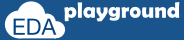# Randomization Methods

SystemVerilog randomization provides a built-in method randomize. The randomize() method generates random values for all the active random variables of an object, subject to the active constraints. Variables declared with the rand keyword will get random values on the object.randomize() method call.The randomize() method returns 1 if the randomization is successful i.e on randomization it's able to assign random values to all the random variables, otherwise, it returns 0.
randomize method associated with below callbacks,
• pre_randomize
• post_randomize

## pre randomize and post randomize methods

• On calling randomize(), pre_randomize() and post_randomize() functions will get called before and after the randomize call respectively
• Users can override the pre_randomize() and post_randomize() functions

### pre_randomize

the pre_randomize function can be used to set pre-conditions before the object randomization.

For example, Users can implement randomization control logic in pre_randomize function. i.e randomization enable or disable by using rand_mode() method.

### post_randomize

the post_randomization function can be used to check and perform post-conditions after the object randomization.

For example, Users can override the randomized values or can print the randomized values of variables.

## randomization method examples

### Implementing pre and post randomize methods

In the below example,
pre and post randomized methods are implemented in the class, on calling obj.randomize() pre and post will get called.

```class packet;
randc bit [7:0] data;

//pre randomization function
function void pre_randomize();
\$display("Inside pre_randomize");
endfunction

//post randomization function
function void post_randomize();
\$display("Inside post_randomize");
endfunction
endclass

module rand_methods;
initial begin
packet pkt;
pkt = new();
pkt.randomize();
end
endmodule
```

Simulator Output

Inside pre_randomize
Inside post_randomize
value of addr = 110, data = 129

Execute the above code on### randomization control from pre_randomize method

In the example below,
Paket has two variables, addr, and wr_rd.

assuming wr_rd = 0 read operation.
wr_rd = 1 write operation.

In order to perform write followed by reading to the same addr, randomization of addr is controlled based on the previous randomization value of wr_rd. this controlling is done in pre_randomize() function.

```//class
class packet;
randc bit       wr_rd;
bit       tmp_wr_rd;

//pre randomization function - disabling randomization of addr,
//if the prevoius operation is write.
function void pre_randomize();
```    else                 addr.rand_mode(1);
endfunction

//post randomization function - store the wr_rd value to tmp_wr_rd
//and display randomized values of addr and wr_rd
function void post_randomize();
tmp_wr_rd = wr_rd;
endfunction
endclass

module rand_methods;
initial begin
packet pkt;
pkt = new();

repeat(4) pkt.randomize();
end
endmodule```

Simulator Output

POST_RANDOMIZATION:: Addr = 6e,wr_rd = 1
POST_RANDOMIZATION:: Addr = 6e,wr_rd = 0
POST_RANDOMIZATION:: Addr = 88,wr_rd = 1
POST_RANDOMIZATION:: Addr = 88,wr_rd = 0

Execute the above code on